# Schwarz alternating method

(diff) ← Older revision | Latest revision (diff) | Newer revision → (diff)

One of the general methods for finding a solution to the Dirichlet problem; it allows one to obtain a solution to the Dirichlet problem for a differential equation of elliptic type in domainsthat can be represented as the union of a finite number of domainsin which the solution to the Dirichlet problem is already known. Studies of H.A. Schwarz (1869; see ) and a number of later studies by other authors were dedicated to this method for finding a solution to the Dirichlet problem for the Laplace equation in plane domains. The principal idea of the Schwarz alternating method as applied to the simplest case of the Laplace equation in the union of two plane domains is the following.

Letandbe two domains in the plane with non-empty intersection and such that the solution to the Dirichlet problem for the Laplace equation is known for each of them. For instance, ifandare discs, then the solution to the Dirichlet problem for each of them is given by the Poisson integral. Further, letbe the union ofandfor which a solution to the Dirichlet problem is sought (see Fig.). Letdenote the boundary of, let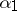denote the parts ofthat are in(they are interior in) and letbe the remaining parts, so that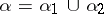. Similarly,is the boundary of,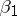are its parts that fall in(they are also interior in) and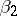are the remaining parts, that is,. Then the boundaryofcan be represented in the form.Figure: s083490a

Now, given a continuous functionon, one has to find a harmonic function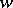inthat is continuous in the closed domain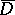and that takes onthe values of. The restriction ofto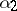can be continuously extended to the whole boundary, and for these boundary values one finds a solution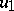to the Dirichlet problem in. The values ofontogether with the values ofonnow form a continuous function onfor which a solutionto the Dirichlet problem inis found. Further, a solution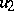to the Dirichlet problem inis constructed, based on the values ofonand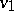on, etc. The sought function has the form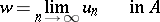and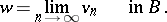Using bounded solutions of the Dirichlet problem with piecewise-continuous boundary data allows one to choose the values zero on the remaining parts of the boundaries without having to worry about the continuous extension of.

A method analogous to the Schwarz alternating method (see ) can be applied to finding a solution to the Dirichlet problem in the intersection of two domainsand, if its solutions forandare known.

Schwarz' alternating method is also used to solve boundary value problems of a more general nature for general equations of elliptic type (including equations of an order greater than two) under certain additional conditions , and also in domains in space.

Schwarz' alternating method is extremely important for the construction of various harmonic functions (with pre-assigned singularities) on Riemann surfaces .